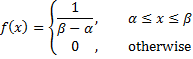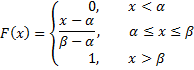# Uniform Distribution

When you ask for a random set of say 100 numbers between 1 and 10, you are looking for a sample from a continuous uniform distribution, where α = 1 and β = 10 according to the following definition.

Definition 1: The continuous uniform distribution has probability density function (pdf) given bywhere α and β are any parameters with α < β.

Observation: The corresponding cumulative distribution function (cdf) isThe inverse cumulative distribution function is

I(p) = α + p(β − α)

Other key statistical properties are:

• Mean = (α + β) / 2
• Median = (α + β) / 2
• Mode = any xα ≤ x ≤ β
• Range = (-∞, ∞)
• Variance = (β – α)2 / 12
• Skewness = 0
• Kurtosis = -1.2

Real Statistics Functions: Excel doesn’t provide any functions for the uniform distribution. Instead you can use the following functions provided by the Real Statistics Resource Pack.

UNIFORM_DIST(x, α, β, cum) = the pdf of the continuous uniform distribution f(x) at x when cum = FALSE and the corresponding cumulative distribution function F(x) when cum = TRUE.

UNIFORM_INV(p, α, β) = x such that UNIFORM_DIST(x, α, β, TRUE) = p. Thus UNIFORM_INV is the inverse of the cumulative distribution version of UNIFORM_DIST.

Statistics with R for Business Analysts – Normal Distribution

# Personal Career & Learning Guide for Data Analyst, Data Engineer and Data Scientist

## Applied Machine Learning & Data Science Projects and Coding Recipes for Beginners

A list of FREE programming examples together with eTutorials & eBooks @ SETScholars

# Projects and Coding Recipes, eTutorials and eBooks: The best All-in-One resources for Data Analyst, Data Scientist, Machine Learning Engineer and Software Developer

Topics included: Classification, Clustering, Regression, Forecasting, Algorithms, Data Structures, Data Analytics & Data Science, Deep Learning, Machine Learning, Programming Languages and Software Tools & Packages.
(Discount is valid for limited time only)`Disclaimer: The information and code presented within this recipe/tutorial is only for educational and coaching purposes for beginners and developers. Anyone can practice and apply the recipe/tutorial presented here, but the reader is taking full responsibility for his/her actions. The author (content curator) of this recipe (code / program) has made every effort to ensure the accuracy of the information was correct at time of publication. The author (content curator) does not assume and hereby disclaims any liability to any party for any loss, damage, or disruption caused by errors or omissions, whether such errors or omissions result from accident, negligence, or any other cause. The information presented here could also be found in public knowledge domains.`

# Learn by Coding: v-Tutorials on Applied Machine Learning and Data Science for Beginners

Please do not waste your valuable time by watching videos, rather use end-to-end (Python and R) recipes from Professional Data Scientists to practice coding, and land the most demandable jobs in the fields of Predictive analytics & AI (Machine Learning and Data Science).

The objective is to guide the developers & analysts to “Learn how to Code” for Applied AI using end-to-end coding solutions, and unlock the world of opportunities!